Win up to 100% scholarship on Aakash BYJU'S JEE/NEET courses with ABNAT Win up to 100% scholarship on Aakash BYJU'S JEE/NEET courses with ABNAT

# JEE Main 2022 July 28 – Shift 1 Maths Question Paper with Solutions

The JEE Main 2022 July 28 – Shift 1 Maths Question Paper with Solutions is given on this page. JEE Main 2022 answer keys are prepared by JEE specialists at BYJU’S. Students can solve these JEE Main 2022 question papers and find out the topics which they need to learn more. It will also help them to boost their confidence. JEE Main 2022 question paper with solutions is a perfect tool to crack the upcoming entrance exams. Go through the JEE Main 2022 July 28 – Shift 1 Maths Question Paper with Solutions given below.

## JEE Main 2022 28th July Shift 1 Mathematics Question Paper and Solutions

SECTION – A

Multiple Choice Questions: This section contains 20 multiple choice questions. Each question has 4 choices (1), (2), (3) and (4), out of which ONLY ONE is correct.

1. Let the solution curve of the differential equation

$$\begin{array}{l}xdy=\left(\sqrt{x^2 + y^2 }+y\right)dx, x>0\end{array}$$
intersect the line x = 1 at y = 0 and the line x = 2 at y = α. Then the value of α is

$$\begin{array}{l}(\text{A})\ \frac{1}{2}\end{array}$$
$$\begin{array}{l}(\text{B})\ \frac{3}{2}\end{array}$$
$$\begin{array}{l}(\text{C})\ -\frac{3}{2}\end{array}$$
$$\begin{array}{l}(\text{D})\ \frac{5}{2}\end{array}$$

Sol.

$$\begin{array}{l}\frac{xdy-ydx}{\sqrt{x^2+y^2}}=dx\end{array}$$
$$\begin{array}{l}\Rightarrow \frac{dy}{dx}=\frac{\sqrt{x^2+y^2}}{x}+\frac{y}{x}\end{array}$$
$$\begin{array}{l}\Rightarrow \frac{dy}{dx}= \sqrt{1+\frac{y^2}{x^2}} +\frac{y}{x}\end{array}$$
$$\begin{array}{l}\text{Let }\frac{y}{x} = v\end{array}$$
$$\begin{array}{l}\Rightarrow v + x\frac{dv}{dx}=\sqrt{1+v^2}+v\end{array}$$
$$\begin{array}{l}\Rightarrow \frac{dv}{\sqrt{1+v^2}}=\frac{dx}{x}\end{array}$$
$$\begin{array}{l}\text{OR}\ \ln \left(v+\sqrt{1+v^2}\right)=\ln x + C\end{array}$$

at x = 1, y = 0

C = 0

$$\begin{array}{l}\frac{y}{x}+\sqrt{1+\frac{y^2}{x^2}}=x\end{array}$$

At x = 2,

$$\begin{array}{l}\frac{y}{2}+\sqrt{1+\frac{y^2}{4}}=2\end{array}$$
$$\begin{array}{l}\Rightarrow 1 + \frac{y^2}{4}=4+\frac{y^2}{4}-2y\end{array}$$
$$\begin{array}{l}\text{OR } y = \frac{3}{2}\end{array}$$

2. Considering only the principal values of the inverse trigonometric functions, the domain of the function

$$\begin{array}{l}f(x)=\cos^{-1}\left(\frac{x^2-4x+2}{x^2+3}\right)\end{array}$$
is

$$\begin{array}{l}(\text{A})\ \left(-\infty, \frac{1}{4}\right]\end{array}$$
$$\begin{array}{l}(\text{B})\ \left[-\frac{1}{4}, \infty \right)\end{array}$$
$$\begin{array}{l}(\text{C})\ \left(\frac{-1}{3}, \infty \right)\end{array}$$
$$\begin{array}{l}(\text{C})\ \left(-\infty, \frac{1}{3} \right]\end{array}$$

Sol.

$$\begin{array}{l}-1\le \frac{x^2-4x+2}{x^2+3}\le1\end{array}$$
$$\begin{array}{l}\Rightarrow -x^2 – 3 \le x^2 – 4x + 2 \le x^2 + 3 \end{array}$$

⇒ 2x2 – 4x + 5 ≥ 0 & -4x ≤ 1

x ∈ R & x ≥ -1/4 ]

$$\begin{array}{l}\text{So domain is }\left[-\frac{1}{4}, \infty\right)\end{array}$$

3. Let the vectors

$$\begin{array}{l}\vec{a}=(1+t)\hat{i}+(1-t)\hat{j}+\hat{k},\ \vec{b}=(1-t)\hat{i}+(1+t)\hat{j}+2\hat{k}\end{array}$$
and
$$\begin{array}{l}\vec{c}=t\hat{i}-t\hat{j}+\hat{k}, t\in \mathbf{R}\end{array}$$
be such that for
$$\begin{array}{l}\alpha, \beta, \gamma \in \mathbf R,\ \alpha \vec{a}+\beta \vec{b}+\gamma \vec{c} =\vec{0}\ \Rightarrow \alpha =\beta = \gamma =0.\end{array}$$
Then, the set of all values of t is

(A) A non-empty finite set

(B) Equal to N

$$\begin{array}{l}\left(C\right) \text{Equal to}~ \mathbf{R} – \{0\}\end{array}$$
$$\begin{array}{l}\left(C\right) \text{Equal to}~ \mathbf{R}\end{array}$$

Sol.

$$\begin{array}{l}\text{Clearly }\vec{a}, \vec{b},\vec{c} \text{ are non-coplanar}\end{array}$$
$$\begin{array}{l}\begin{vmatrix} 1+t& 1-t & 1 \\1-t & 1+t & 2 \\t & -t & 1 \\\end{vmatrix} \ne 0\end{array}$$
$$\begin{array}{l}\Rightarrow \left(1 + t\right)\left(1 + t + 2t\right) – \left(1 – t\right)\left(1 – t – 2t\right)+ 1\left(t^2 – t – t – t^2\right) \ne 0\end{array}$$
$$\begin{array}{l}\Rightarrow \left(3t^2 + 4t + 1\right) – \left(1 – t\right) \left(1 – 3t\right) – 2t \neq 0\end{array}$$
$$\begin{array}{l}\Rightarrow \left(3t^2 + 4t + 1\right) – \left(3t^2 – 4t + 1\right) – 2t \neq 0\end{array}$$
$$\begin{array}{l}\Rightarrow t \neq 0\end{array}$$

4. Considering the principal values of the inverse trigonometric functions, the sum of all the solutions of the equation

$$\begin{array}{l}cos^{-1}\left(x\right) – 2sin^{-1}\left(x\right) = cos^{-1}\left(2x\right)\end{array}$$
is equal to

$$\begin{array}{l}(\text{A})0\end{array}$$
$$\begin{array}{l}(\text{B})1\end{array}$$
$$\begin{array}{l}(\text{C})\ \frac{1}{2}\end{array}$$
$$\begin{array}{l}(\text{D})\ -\frac{1}{2}\end{array}$$

Sol.

$$\begin{array}{l}cos^{-1}x – 2sin^{-1}x = cos^{-1}2x\end{array}$$
$$\begin{array}{l}\text{For Domain :}x \in \left[\frac{-1}{2},\frac{1}{2}\right]\end{array}$$
$$\begin{array}{l}\cos^{-1}x-2\left(\frac{\pi}{2}-\cos^{-1}x\right)=\cos^{-1}(2x)\end{array}$$
$$\begin{array}{l} \Rightarrow cos^{-1}x + 2cos^{-1}x = \pi + cos^{-1}2x\end{array}$$
$$\begin{array}{l}\Rightarrow cos\left(3cos^{–1}x\right) = – cos\left(cos^{–1}2x\right)\end{array}$$
$$\begin{array}{l}\Rightarrow 4x^3 = x\end{array}$$
$$\begin{array}{l}\Rightarrow x = 0, \pm \frac{1}{2}\end{array}$$

5. Let the operations *, ◉ ∈ {∧, ∨}. If (p * q) ◉ (p ◉ ~q) is a tautology, then the ordered pair (*, ◉) is

(A) (∨, ∧)

(B) (∨, ∨)

(C) (∧, ∧)

(D) (∧, ∨)

Sol. *, ◉ ∈ {∧, ∨}.

Now for (q * q)◉(p◉~q) is tautology

(A) (∨, ∧) : (pq) ∧ (p ∧ ~q) not a tautology

(B) (∨, ∨) : (pq) ∨ (p ∨ ~q)

= PT is tautology

(C) (∧ , ∧) : (pq) ∧ (p ∧ ~q)

= (pp) ∧ (q ∧ ~q) = pF not a tautology (Fallasy)

(D) (∧, ∨) : (pq) ∨ (p ∨ ~q) not a tautology

6. Let a vector

$$\begin{array}{l}\vec{a}\ \text{has magnitude}\ 9.\ \text{Let a vector}\ \vec{b}\end{array}$$
be such that for every
$$\begin{array}{l}\left(x, y\right) \in \mathbf R \times \mathbf R – \{\left(0, 0\right)\},\ \text{the vector}\ (x\vec{a} + y\vec{b})\end{array}$$

$$\begin{array}{l}\text{is perpendicular to the vector}\ (6y\vec{a} – 18x\vec{b}).\end{array}$$
$$\begin{array}{l}\text{Then the value of}\ |\vec{a}\times \vec{b}|\end{array}$$
is equal to

$$\begin{array}{l}(\text{A})\ 9\sqrt{3}\end{array}$$
$$\begin{array}{l}(\text{B})\ 27\sqrt{3}\end{array}$$
$$\begin{array}{l}(\text{C})\ 9\end{array}$$
$$\begin{array}{l}(\text{D})\ 81\end{array}$$

Sol.

$$\begin{array}{l}(x\vec{a} + y\vec{b})\cdot(6y\vec{a}-18x\vec{b})=0\end{array}$$
$$\begin{array}{l}\Rightarrow \left(6xy|\vec{a}|^2 – 18 xy|\vec{b}|^2\right)+\left(6y^2 -18x^2\right)\vec{a}.\vec{b}=0\end{array}$$

As given equation is identity

Coefficient of x2 = coefficient of y2 = coefficient of
xy = 0

$$\begin{array}{l}\Rightarrow |\vec{a}|^2 = 3|\vec{b}|^2 \Rightarrow |\vec{b}| = 3\sqrt{3}\end{array}$$
$$\begin{array}{l}\text{and } \vec{a}.\vec{b}=0\end{array}$$
$$\begin{array}{l}|\vec{a}\times \vec{b}| = |\vec{a}|| \vec{b}|\sin \theta\end{array}$$
$$\begin{array}{l}=9.3\sqrt{3}.1=27\sqrt{3}\end{array}$$

7. For t ∈ (0, 2π), if ABC is an equilateral triangle with vertices A(sint, – cost), B(cost, sint) and C(a, b) such that its orthocentre lies on a circle with centre (1, 1/3), then (a2 – b2) is equal to

$$\begin{array}{l}(\text{A})\ \frac{8}{3}\end{array}$$
$$\begin{array}{l}(\text{B})\ 8\end{array}$$
$$\begin{array}{l}(\text{C})\ \frac{77}{9}\end{array}$$
$$\begin{array}{l}(\text{D})\ \frac{80}{9}\end{array}$$

Sol. Let P(h, k) be the orthocentre of ΔABC.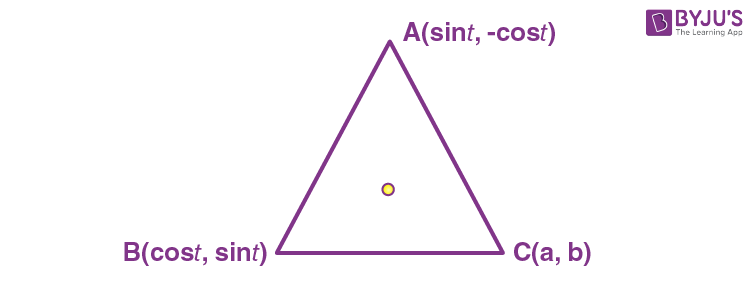Then

$$\begin{array}{l}h=\frac{\sin t + \cos t + a}{3}, k= \frac{-\cos t + \sin t + b}{3}\end{array}$$

(orthocentre coincide with centroid)

$$\begin{array}{l}\therefore \left(3h – a\right)^2 + \left(3k – b\right)^2 = 2\end{array}$$
$$\begin{array}{l}\therefore \left(h-\frac{a}{3}\right)^2 + \left(k-\frac{b}{3}\right)^2 = \frac{2}{9}\end{array}$$
$$\begin{array}{l}\because\end{array}$$
orthocentre lies on circle with centre
$$\begin{array}{l}\left(1, \frac{1}{3}\right)\end{array}$$

∴ a = 3, b = 1

∴ a2 – b2 = 8

8. For α ∈ N, consider a relation R on N given by R = {(x, y) : 3x + αy is a multiple of 7}. The relation R is an equivalence relation if and only if

(A) α = 14

(B) α is a multiple of 4

(C) 4 is the remainder when α is divided by 10

(D) 4 is the remainder when α is divided by 7

Sol.

$$\begin{array}{l}R = \{(x, y) : 3x + \alpha y~ \text{is a multiple of} ~7\}\end{array}$$
, Now R to be an equivalence relation

$$\begin{array}{l}\left(1\right) \text{R should be reflexive }: \left(a, a\right)\in R~ \forall a \in N\\ \therefore 3a + a \alpha = 7k\end{array}$$
$$\begin{array}{l}\therefore \left(3 + \alpha \right) a = 7k\end{array}$$
$$\begin{array}{l}\therefore 3 + \alpha = 7k_1 \Rightarrow \alpha = 7k_1 – 3\\ = 7k_1 + 4\end{array}$$
$$\begin{array}{l}\left(2\right) R~ \text{should be symmetric} : aRb \Leftrightarrow bRa \end{array}$$
$$\begin{array}{l}aRb : 3a + \left(7k -3\right) b = 7 m \end{array}$$
$$\begin{array}{l}\Rightarrow 3\left(a – b\right) + 7kb = 7 m\\\Rightarrow 3\left(b – a\right) + 7 ka = 7 m\end{array}$$
$$\begin{array}{l}So, aRb \Rightarrow bRa\end{array}$$
$$\begin{array}{l}\therefore \text{R will be symmetric for}~ a = 7k_1 – 3 \end{array}$$
$$\begin{array}{l}\left(3\right) \text{Transitive : Let} \left(a, b\right)\in R, (b, c)\in R\end{array}$$
$$\begin{array}{l}\Rightarrow 3a + \left(7k – 3\right)b = 7k_1 ~\text{and}~\\ 3b + \left(7k_2 – 3\right) c = 7k_3\end{array}$$

Adding 3a + 7kb + (7k2 – 3) c = 7 (k1 + k3)

3a + (7k2 – 3) c = 7 m

$$\begin{array}{l}\therefore \left(a, c\right)\in R\end{array}$$

R is transitive

$$\begin{array}{l}\therefore a = 7k – 3 = 7k + 4\end{array}$$

9. Out of 60% female and 40% male candidates appearing in an exam, 60% of candidates qualify it. The number of females qualifying the exam is twice the number of males qualifying it. A candidate is randomly chosen from the qualified candidates. The probability that the chosen candidate is a female, is

$$\begin{array}{l}(\text{A})\ \frac{3}{4}\end{array}$$
$$\begin{array}{l}(\text{B})\ \frac{11}{16}\end{array}$$
$$\begin{array}{l}(\text{C})\ \frac{23}{32}\end{array}$$
$$\begin{array}{l}(\text{D})\ \frac{13}{16}\end{array}$$

Answer (*) None of the given option is correct

Sol.

$$\begin{array}{l}P(\text{Female})= \frac{60}{100}=\frac{3}{5}\end{array}$$
$$\begin{array}{l}P(\text{Male})= \frac{2}{5}\end{array}$$
$$\begin{array}{l}P(\text{Female/Qualified})= \frac{40}{60} = \frac{2}{3}\end{array}$$
$$\begin{array}{l}P(\text{Male/Qualified})= \frac{20}{60} = \frac{1}{3}\end{array}$$

10. If y = y(x), x ∈ (0, π/2) be the solution curve of the differential equation

$$\begin{array}{l}(\sin^22x)\frac{dy}{dx}+ (8\sin^22x + 2\sin 4x)y = 2e^{-4x}(2\sin 2x + \cos 2x),\end{array}$$
$$\begin{array}{l}\text{with}\ y\left(\frac{\pi}{4}\right)=e^{-\pi},\ \text{then}\ y\left(\frac{\pi}{6}\right)\end{array}$$
is equal to

$$\begin{array}{l}(\text{A})\ \frac{2}{\sqrt{3}}e^{-2\pi/3}\end{array}$$
$$\begin{array}{l}(\text{B})\ \frac{2}{\sqrt{3}}e^{2\pi/3}\end{array}$$
$$\begin{array}{l}(\text{C})\ \frac{1}{\sqrt{3}}e^{-2\pi/3}\end{array}$$
$$\begin{array}{l}(\text{D})\ \frac{1}{\sqrt{3}}e^{2\pi/3}\end{array}$$

Sol.

$$\begin{array}{l}(\sin^22x)\frac{dy}{dx}+(8\sin^2 2x + 2\sin 4x)y=2e^{-4x}(2\sin 2x + \cos 2x)\end{array}$$
$$\begin{array}{l}\frac{dy}{dx}+(8+4\cot 2x)y = 2e^{-4x}\left(\frac{2\sin 2x + \cos 2x}{\sin^2 2x}\right)\end{array}$$
$$\begin{array}{l} \text{Integrating factor} (I.F.)=e^{\int (8+4\cot 2x)dx}\end{array}$$
$$\begin{array}{l}=e^{8x+2\ln \sin 2x}\end{array}$$

Solution of differential equation

$$\begin{array}{l}y.e^{8x+2\ln \sin 2x} = \int 2e^{(4x + 2\ln \sin2x)}\frac{(2\sin 2x + \cos 2x)}{\sin^2 2x}dx\end{array}$$
$$\begin{array}{l}=2\int e^{4x}(2 \sin 2x + \cos 2x)dx\end{array}$$
$$\begin{array}{l}y.e^{8x + 2\ln \sin 2x}=e^{4x}\sin 2x + c\end{array}$$
$$\begin{array}{l}y\left(\frac{\pi}{4}\right)=e^{-\pi}\end{array}$$
$$\begin{array}{l}e^{-\pi}.e^{2\pi}=e^{\pi}+c \Rightarrow c=0\end{array}$$
$$\begin{array}{l}y\left(\frac{\pi}{6}\right)=\frac{e^{\frac{2\pi}{3}}\frac{\sqrt{3}}{2}}{e^{\left(\frac{4\pi}{3}+2\ln \frac{\sqrt{3}}{2}\right)}}\end{array}$$
$$\begin{array}{l}=e^{\frac{-2\pi}{3}}.\frac{2}{\sqrt{3}}\end{array}$$

11. If the tangents drawn at the points P and Q on the parabola y2 = 2x – 3 intersect at the point R(0, 1), then the orthocentre of the triangle PQR is :

(A) (0, 1)

(B) (2, –1)

(C) (6, 3)

(D) (2, 1)

Sol.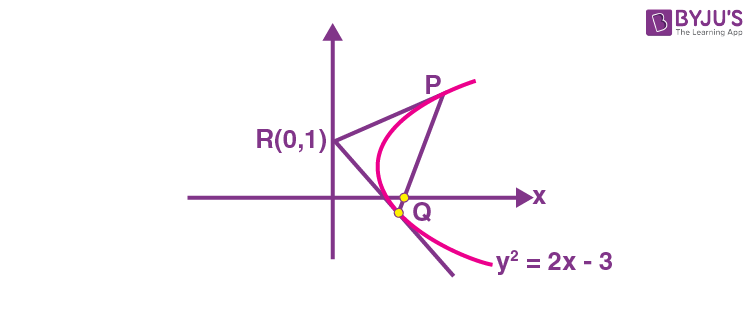Equation of chord PQ

y × 1 = x – 3

xy = 3

For point P & Q

Intersection of PQ with parabola P : (6, 3) Q : (2, –1)

Slope of RQ = –1 & Slope of PQ = 1

$$\begin{array}{l}\text{Therefore}~ \angle PQR = 90 ^\circ \Rightarrow \text{Orthocentre is at} Q : \left(2, -1\right)\end{array}$$

12. Let C be the centre of the circle

$$\begin{array}{l}x^2+y^2-x+2y=\frac{11}{4}\end{array}$$
and P be a point on the circle. A line passes through the point C, makes an angle of π/4with the line CP and intersects the circle at the Q and R. Then the area of the triangle PQR (in unit2) is :

$$\begin{array}{l}(\text{A})\ 2\end{array}$$
$$\begin{array}{l}(\text{B})\ 2\sqrt{2}\end{array}$$
$$\begin{array}{l}(\text{C})\ 8\sin\left(\frac{\pi}{8}\right)\end{array}$$
$$\begin{array}{l}(\text{D})\ 8\cos\left(\frac{\pi}{8}\right)\end{array}$$

Sol.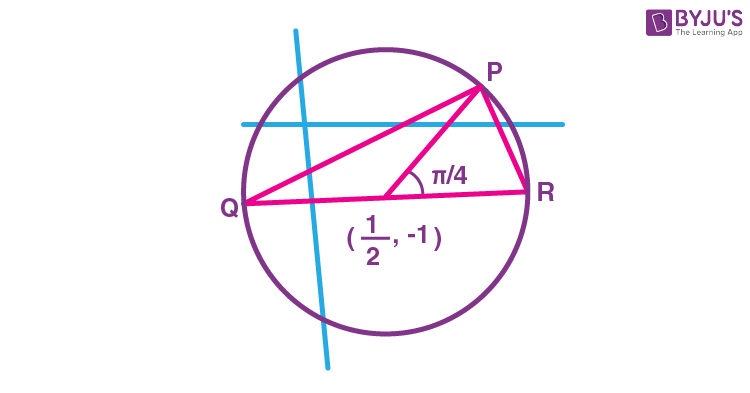QR = 2r = 4[

$$\begin{array}{l}P=\left(\frac{1}{2}+2\cos\frac{\pi}{4}, -1 + 2\sin \frac{\pi}{4}\right)\end{array}$$
$$\begin{array}{l}=\left(\frac{1}{2}+\sqrt{2},-1 + \sqrt{2} \right)\end{array}$$
$$\begin{array}{l}\text{Area of } \Delta PQR = \frac{1}{2}\times 4 \times \sqrt{2}\end{array}$$
$$\begin{array}{l}=2\sqrt{2} \text{ sq. units}\end{array}$$

13. The remainder 72022 + 32022 is divided by 5 is:

(A) 0

(B) 2

(C) 3

(D) 4

Sol. Let E = 72022 + 32022

= (15 – 1)1011 + (10 – 1)1011

= –1 + (multiple of 15) –1 + multiple of 10

= –2 + (multiple of 5)

Hence remainder on dividing E by 5 is 3.

14. Let the matrix

$$\begin{array}{l}A= \begin{vmatrix}0 & 1 & 0 \\0 & 0 & 1 \\1 & 0 & 0 \\\end{vmatrix}\end{array}$$
and the matrix
$$\begin{array}{l}B_0 = A^{49} + 2A^{98}. ~\text{If} ~B_n = Adj(B_{n – 1})~\text{for all}~n \geq 1,\end{array}$$
then det(B4) is equal to :

(A) 328

(B) 330

(C) 332

(D) 336

Sol.

$$\begin{array}{l}A= \begin{bmatrix}0 & 1 & 0 \\0 & 0 & 1 \\1 & 0 & 0 \\\end{bmatrix}\end{array}$$
$$\begin{array}{l}\Rightarrow A^2= \begin{bmatrix}0 & 1 & 0 \\0 & 0 & 1 \\1 & 0 & 0 \\\end{bmatrix} \times \begin{bmatrix}0 & 1 & 0 \\0 & 0 & 1 \\1 & 0 & 0 \\\end{bmatrix} = \begin{bmatrix}0 & 0 & 1 \\1 & 0 & 0 \\0 & 1 & 0 \\\end{bmatrix}\end{array}$$
$$\begin{array}{l}\Rightarrow A^3= \begin{bmatrix}0 & 0 & 1 \\1 & 0 & 0 \\0 & 1 & 0 \\\end{bmatrix} \begin{bmatrix}0 & 1 & 0 \\0 & 0 & 1 \\1 & 0 & 0 \\\end{bmatrix} = \begin{bmatrix}1 & 0 & 0 \\0 & 1 & 0 \\0 & 0 & 1 \\\end{bmatrix} = I\end{array}$$
$$\begin{array}{l}\text{Now } B_0 = A^{49}+ 2A^{98}=\left(A^3\right)^{16}.A+2\left(A^3\right)^{32}.A^2\end{array}$$
$$\begin{array}{l}B_0=A+2A^2= \begin{bmatrix}0 & 1 & 0 \\0 & 0 & 1 \\1 & 0 & 0 \\\end{bmatrix}+ \begin{bmatrix}0 & 0 & 2 \\2 & 0 & 0 \\0 & 2 & 0 \\\end{bmatrix} = \begin{bmatrix}0 & 1 & 2 \\2 & 0 & 1 \\1 & 2 & 0 \\\end{bmatrix}\end{array}$$
$$\begin{array}{l}\left|B_0\right|= 9 \end{array}$$
$$\begin{array}{l}\text{Since}, B_n = Adj \left|B_{n – 1}\right|\Rightarrow \left|B_n\right| = \left|B_{n – 1}\right|^2 \end{array}$$
$$\begin{array}{l}\text{Hence} \left|B_4\right| = \left|B_3\right|^2 = \left|B_2\right|^4 = \left|B_1\right|^8 = \left|B_0\right|^{16} = \left|3^2\right|^{16} = 3^{32}\end{array}$$

15. Let

$$\begin{array}{l}S_1= \left\{z_1 \in C : |z_1 – 3| = \frac{1}{2}\right\}\ \text{and}\ S_2= \left\{z_2 \in C : |z_2 – |z_2 + 1|| = |z_2 + |z_2 – 1||\right\}.\end{array}$$
Then, for z1 ∈ S1 and z2 ∈ S2, the least value of |z2 – z1| is :

$$\begin{array}{l}(\text{A})\ 0\end{array}$$
$$\begin{array}{l}(\text{B})\ \frac{1}{2}\end{array}$$
$$\begin{array}{l}(\text{C})\ \frac{3}{2}\end{array}$$
$$\begin{array}{l}(\text{D})\ \frac{5}{2}\end{array}$$

Sol.

$$\begin{array}{l}\because |Z_2 + |Z_2 -1||^2=|Z_2- |Z_2+1| |^2\end{array}$$
$$\begin{array}{l}\Rightarrow (Z_2 + |Z_2-1|)(\bar{Z}_2 + |Z_2-1|)=(Z_2- |Z_2-1|)(\bar{Z}_2-|Z_2+1|)\end{array}$$
$$\begin{array}{l}\Rightarrow Z_2(|Z_2-1|+|Z_2+1|)+ \bar{Z}_2(|Z_2-1| + |Z_2+1|)=|Z_2 + 1|^2 – |Z_2-1|^2\end{array}$$
$$\begin{array}{l}\Rightarrow (Z_2 + \bar{Z}_2)(|Z_2+1|+|Z_2-1|)=2(Z_2 + \bar{Z}_2)\end{array}$$
$$\begin{array}{l}\Rightarrow \text{Either } Z_2 + \bar{Z}_2 = 0 \text{ or } |Z_2 + 1| + |Z_2 + 1|=2\end{array}$$

So, Z2 lies on imaginary axis or on real axis within [–1, 1]

$$\begin{array}{l} \text{Also} |Z_1-3|=\frac{1}{2}\Rightarrow Z_1\end{array}$$
lies on the circle having center 3 and radius ½.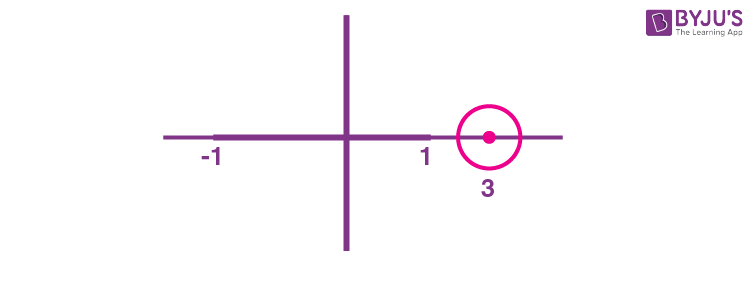$$\begin{array}{l}\text{Clearly } |Z_1 – Z_2|_{\text{min}} = \frac{3}{2}\end{array}$$

16. The foot of the perpendicular from a point on the circle x2 + y2 = 1, z = 0 to the plane 2x + 3y + z = 6 lies on which one of the following curves?

$$\begin{array}{l}\left(A\right) \left(6x + 5y – 12\right)^2 + 4\left(3x + 7y – 8\right)^2 = 1, z = 6 – 2x – 3y\end{array}$$
$$\begin{array}{l}\left(B\right)\left(5x + 6y – 12\right)^2 + 4\left(3x + 5y – 9\right)^2 = 1, z = 6- 2x – 3y\end{array}$$
$$\begin{array}{l}\left(C\right) \left(6x + 5y – 14\right)^2 + 9\left(3x + 5y – 7\right)^2 = 1, z = 6 – 2x – 3y\end{array}$$
$$\begin{array}{l}\left(D\right) \left(5x + 6y – 14\right)^2 + 9\left(3x + 7y – 8\right)^2 = 1, z = 6 – 2x – 3y\end{array}$$

Sol. Any point on x2 + y2 = 1, z = 0 is p(cosθ, sinθ, 0)

If foot of perpendicular of p on the plane 2x + 3y + z = 6 is (h, k, l) then

$$\begin{array}{l}\frac{h-\cos \theta}{2}=\frac{k-\sin \theta}{3}=\frac{l-0}{1}\end{array}$$
$$\begin{array}{l}=-\left(\frac{2\cos \theta + 3\sin \theta +0 -6}{2^2 + 3^2 + 1^2}\right)=r(\text{let})\end{array}$$

h = 2r + cosθ, k = 3r + sinθ, l = r

Hence, h – 2l = cosθ and k – 3l = sinθ

Hence (h – 2l)2 + (k – 3l)2 = 1

When l = 6 – 2h – 3k

Hence required locus is

$$\begin{array}{l}\left(x – 2\left(6 – 2x – 3y\right)\right)^2 + \left(y – 3\left(6 – 2x – 3y\right)\right)^2 = 1\end{array}$$
$$\begin{array}{l}\Rightarrow \left(5x + 6y – 12\right)^2 + 4\left(3x + 5y – 9\right)^2 = 1, z = 6 – 2x – 3y\end{array}$$

17. If the minimum value of

$$\begin{array}{l}f(x)=\frac{5x^2}{2}+\frac{\alpha}{x^5}, x>0\end{array}$$
is 14, then the value of α is equal to

(A) 32

(B) 64

(C) 128

(D) 256

Sol.

$$\begin{array}{l}f(x)=\frac{5x^2}{2}+\frac{\alpha}{x^5} \ \ \{x>0\}\end{array}$$
$$\begin{array}{l}f'(x)=5x-\frac{5\alpha}{x^6}=0\end{array}$$$$\begin{array}{l}\Rightarrow x = (\alpha)^{1/7}\end{array}$$
$$\begin{array}{l}f(x)_{\text{min}}=\frac{5(\alpha)^{2/7}}{2}+\frac{\alpha}{a^{5/7}}=14\end{array}$$
$$\begin{array}{l}\frac{5}{2}\alpha^{2/7}+\alpha^{2/7}=14\end{array}$$
$$\begin{array}{l}\frac{7}{2}\alpha^{2/7} = 14\\ \alpha = 128\end{array}$$

18. Let α, β and γ be three positive real numbers.

$$\begin{array}{l}Let f\left(x\right) = \alpha x^5 + \beta x^3 + \gamma x,x \in \mathbb {R} ~\text{and } g:\mathbb{R} \rightarrow \mathbb{R}~\text{be such that}~ g\left(f(x)\right) = x~\text{for all}~ x \in \mathbb{R}. \end{array}$$
If a1, a2, a3, …, an be in arithmetic progression with mean zero, then the value of
$$\begin{array}{l}f\left( g\left( \frac{1}{n} \sum_{i=1}^{n}f(a_i)\right)\right)\end{array}$$
is equal to

(A) 0

(B) 3

(C) 9

(D) 27

Sol.

$$\begin{array}{l}f\left( g\left( \frac{1}{n} \sum_{i=1}^{n}f(a_i)\right)\right)\end{array}$$
$$\begin{array}{l}\frac{a_1+a_2+a_3+…+a_n}{n}=0\end{array}$$

∴ First and last term, second and second last and so on are equal in magnitude but opposite in sign.

$$\begin{array}{l}f(x)=\alpha x^5 + \beta x^3 + \gamma x\end{array}$$
$$\begin{array}{l}\sum_{i=1}^{n}f(a_i)=\alpha\left(a_1^5 + a_2^5 + a_3^5 + … + a_n^5\right) + \beta\left(a_1^3 + a_2^3 + … + a_n^3\right) + \gamma\left(a_1+a_2 + … + a_n\right)\end{array}$$
$$\begin{array}{l}= 0\alpha + 0\beta + 0\gamma= 0\end{array}$$
$$\begin{array}{l}\therefore f\left(g\left(\frac{1}{n}\sum^{n}_{i=1}f\left(a_i\right)\right)\right)= \frac{1}{n}\sum^{n}_{i=1}f\left(a_i\right)=0\end{array}$$

19. Consider the sequence a1, a2, a3, … such that a1 = 1, a2 = 2 and

$$\begin{array}{l}a_{n+2}=\frac{2}{a_{n+1}}+a_n \text{ for } n = 1,2,3,…\end{array}$$
. If
$$\begin{array}{l}\left(\frac{a_1+\frac{1}{a_2}}{a_3}\right)\left(\frac{a_2+\frac{1}{a_3}}{a_4}\right)\left(\frac{a_3+\frac{1}{a_4}}{a_5}\right)\cdots\left(\frac{a_{30}+\frac{1}{a_{31}}}{a_{32}}\right)=2^\alpha (^{61}C_{31}), \end{array}$$
then α is equal to

(A) –30

(B) –31

(C) –60

(D) –61

Sol.

$$\begin{array}{l}a_{n+2}=\frac{2}{a_{n+1}}+a_n\end{array}$$
$$\begin{array}{l}\Rightarrow a_na_{n+1}+1 = a_{n+1}a_{n+2}-1\end{array}$$
$$\begin{array}{l}\Rightarrow a_{n+2}a_{n+1}+1 – a_n.a_{n+1}=2\end{array}$$

For n = 1 a3a2a1a2 = 2

n = 2 a4a3a3a2 = 2

n = 3 a5a4a4a3 = 2

:

n = nan + 2an + 1anan + 1 = 2an + 2 an + 1 = 2n + a1a2

Now,

$$\begin{array}{l}\frac{(a_1a_2+1)}{a_2a_3}.\frac{(a_2a_3+1)}{a_3a_4}.\frac{(a_3a_4+1)}{a_4a_5}.\ … \ . \frac{(a_{30}a_{31}+1)}{a_{31}a_{32}}\end{array}$$
$$\begin{array}{l}=\frac{3}{4}.\frac{5}{6}.\frac{7}{8}.\ … \ . \frac{61}{62}\end{array}$$
$$\begin{array}{l}=2^{-60}\left(^{61}C_{31}\right)\end{array}$$

20. The minimum value of the twice differentiable function

$$\begin{array}{l}f(x)=\int_{0}^{x}e^{x-t}f'(t)dt-(x^2-x+1)e^x, x\in \mathbb{R}\end{array}$$
is

$$\begin{array}{l}(\text{A}) \ -\frac{2}{\sqrt{e}}\end{array}$$
$$\begin{array}{l}(\text{B}) \ -2\sqrt{e}\end{array}$$
$$\begin{array}{l}(\text{C}) \ -\sqrt{e}\end{array}$$
$$\begin{array}{l}(\text{D}) \ \frac{2}{\sqrt{e}}\end{array}$$

Sol.

$$\begin{array}{l}f(x)=\int_{0}^{x}e^{x-t}f'(t)dt-(x^2-x+1)e^x\end{array}$$
$$\begin{array}{l}f(x)=e^x\int_{0}^{x}e^{-t}f'(t)dt-(x^2-x+1)e^x\end{array}$$
$$\begin{array}{l}e^{-x}f(x)=\int_{0}^{x}e^{-t}f'(t)dt-(x^2-x+1)\end{array}$$

Differentiate on both sides

$$\begin{array}{l}e^{-x}f'(x)+\left(-f(x)e^{-x}\right)=e^{-x}f'(x)-2x+1\end{array}$$
$$\begin{array}{l}f(x)=e^x(2x-1)\end{array}$$
$$\begin{array}{l}f'(x)=e^x(2)+e^x(2x-1)\end{array}$$
$$\begin{array}{l}=e^x(2x+1)\end{array}$$
$$\begin{array}{l}x=-\frac{1}{2}\end{array}$$
$$\begin{array}{l}f”(x)=e^x(2)+(2x+1)e^x\end{array}$$
$$\begin{array}{l}=e^x(2x+3)\end{array}$$
$$\begin{array}{l}\text{For } x= -\frac{1}{2}\ \ f”(x)>0\end{array}$$
$$\begin{array}{l}\Rightarrow {Maxima}\therefore \text{ Max } = e^{-\frac{1}{2}}(-1-1)\end{array}$$
$$\begin{array}{l}\therefore Max.=-\frac{2}{\sqrt{e}}\end{array}$$

SECTION – B

Numerical Value Type Questions: This section contains 10 questions. In Section B, attempt any five questions out of 10. The answer to each question is a NUMERICAL VALUE. For each question, enter the correct numerical value (in decimal notation, truncated/rounded-off to the second decimal place; e.g. 06.25, 07.00, –00.33, –00.30, 30.27, –27.30) using the mouse and the on-screen virtual numeric keypad in the place designated to enter the answer.

1. Let S be the set of all passwords which are six to eight characters long, where each character is either an alphabet from {A, B, C, D, E} or a number from {1, 2, 3, 4, 5} with the repetition of characters allowed. If the number of passwords in S whose at least one character is a number from {1, 2, 3, 4, 5} is α × 56, then α is equal to _______.

Sol. If password is 6 character long, tehn

Total number of ways having atleast one number = 106 – 56

Similarly, if 7 character long = 107 – 57

and if 8-character long = 108 – 58

$$\begin{array}{l}\text{Number of password = }(10^6 + 10^7 + 10^8) – (5^6 + 5^7 + 5^8) \end{array}$$
$$\begin{array}{l}= 5^6 \left(2^6 + 5.2^7 + 25.2^8 – 1 – 5 – 25\right)\end{array}$$
$$\begin{array}{l}= 56\left(64 + 640 + 6400 – 31\right)\end{array}$$
$$\begin{array}{l}= 7073 \times 5^6\\\therefore \alpha = 7073\end{array}$$
.

2. Let P(–2, –1, 1) and Q(56/17, 43/17, 111/17) be the vertices of the rhombus PRQS. If the direction ratios of the diagonal RS are α, –1, β, where both α and β are integers of minimum absolute values, then α2 + β2 is equal to ___________.

Sol.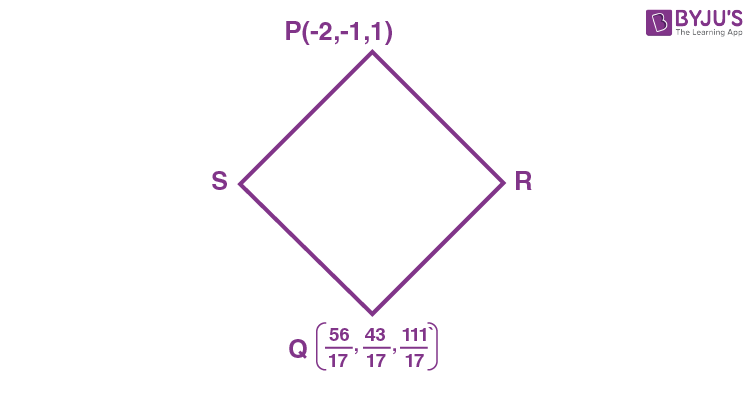d.r’s of RS = < α, –1, β >

$$\begin{array}{l}\text{d.r’s of }PQ = <\frac{90}{17},\frac{60}{17},\frac{94}{17}>=<45, 30,47>\end{array}$$

as PQ and RS are diagonals of rhombus

$$\begin{array}{l} \alpha\left(45\right) + 30\left(-1\right) + 47\left(\beta\right) = 0\\ \Rightarrow 45\alpha + 47\beta = 30\end{array}$$
$$\begin{array}{l}i.e., \alpha = \frac{30-47\beta}{45}\end{array}$$

for minimum integral value α = – 15 and β = 15

$$\begin{array}{l}\Rightarrow \alpha^2 + \beta^2 = 450.\end{array}$$

3. Let f : [0, 1] → R be a twice differentiable function in (0, 1) such that f(0) = 3 and f(1) = 5. If the line
y = 2x + 3 intersects the graph of f at only two distinct points in (0, 1) then the least number of points x ∈ (0, 1) at which f”(x) = 0, is ___________.

Sol.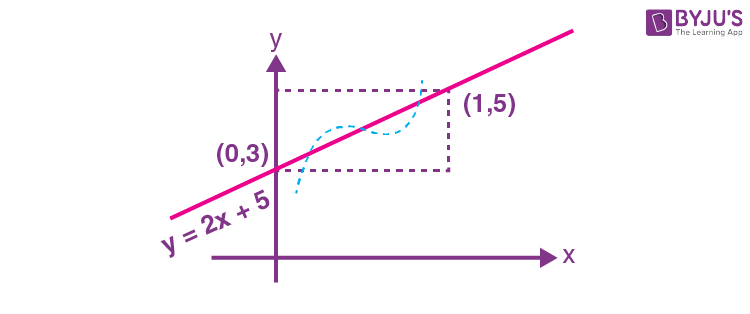If a graph cuts y = 2x + 5 in (0, 1) twice then its concavity changes twice

f”(x) = 0 at atleast two points.

4. If

$$\begin{array}{l}\int_{0}^{\sqrt{3}}\frac{15x^3}{\sqrt{1+x^2 + \sqrt{(1+x^2)^3}}}dx = \alpha \sqrt{2}+\beta\sqrt{3},\end{array}$$
where α, β are integers, then α + β is equal to

Sol. Put

$$\begin{array}{l}x = tan\theta \Rightarrow dx = sec^2\theta~ d\theta\end{array}$$
$$\begin{array}{l}\Rightarrow I = \int_{0}^{\frac{\pi}{3}}\frac{15\tan^3\theta . \sec^2\theta\ d\theta}{\sqrt{1+\tan^2\theta + \sqrt{\sec^6\theta}}}\end{array}$$
$$\begin{array}{l}\Rightarrow I = \int_{0}^{\frac{\pi}{3}}\frac{15\tan^2\theta \sec^2 \theta \ d\theta}{\sec \theta \sqrt{1+ \sec \theta}}\end{array}$$
$$\begin{array}{l}\Rightarrow I = \int_{0}^{\frac{\pi}{3}}\frac{15(\sec^2 \theta -1)\sec \theta \tan \theta \ d\theta}{\left(\sqrt{1+\sec \theta}\right)}\end{array}$$

Now put 1 + secθ = t2

$$\begin{array}{l}\Rightarrow sec~\theta ~tan~\theta~ d\theta = 2tdt\end{array}$$
$$\begin{array}{l}\Rightarrow I = \int_{\sqrt{2}}^{\sqrt{3}}\frac{15((t^2-1)^2-1)2t \ dt}{t}\end{array}$$
$$\begin{array}{l}\Rightarrow I = 30\int_{\sqrt{2}}^{\sqrt{3}}\left(t^4-2t^2 + 1-1\right)dt\end{array}$$
$$\begin{array}{l}\Rightarrow I = 30\int_{\sqrt{2}}^{\sqrt{3}}\left(t^4-2t^2 \right)dt\end{array}$$
$$\begin{array}{l}\Rightarrow I = 30 \left(\frac{t^5}{5}-\frac{2t^3}{3}\right)_{\sqrt{2}}^{\sqrt{3}}\end{array}$$
$$\begin{array}{l}=30\left[\left(\frac{9}{5}\sqrt{3}-2\sqrt{3}\right)-\left(\frac{4\sqrt{2}}{5}-\frac{4\sqrt{2}}{3}\right)\right]\end{array}$$
$$\begin{array}{l}=(54\sqrt{3}-60\sqrt{3})-(24\sqrt{2}-40\sqrt{2})\end{array}$$
$$\begin{array}{l}=16\sqrt{2}-6\sqrt{3}\end{array}$$
$$\begin{array}{l} \therefore \alpha = 16 ~\text{and}~ \beta = – 6\\ \alpha + \beta = 10.\end{array}$$

5. Let

$$\begin{array}{l}A=\begin{bmatrix}1 & -1 \\2 & \alpha \\\end{bmatrix}\ \text{and}\ B=\begin{bmatrix}\beta & 1 \\1 & 0 \\\end{bmatrix},\ alpha, \beta \in R.\end{array}$$
Let α1 be the value of α which satisfies
$$\begin{array}{l}(A + B)^2 =A^2 + \begin{bmatrix}2 & 2 \\2 & 2 \\\end{bmatrix}\end{array}$$
and α2 be the value of α which satisfies
$$\begin{array}{l}\left(A + B\right)^2 = B^2.\end{array}$$
Then |α1 – α2| is equal to _________.

Sol.

$$\begin{array}{l}\left(A + B\right)^2 = A^2 + B^2 + AB + BA\end{array}$$
$$\begin{array}{l}=A^2 + \begin{bmatrix}2 & 2 \\2 & 2 \\\end{bmatrix}\end{array}$$
$$\begin{array}{l}\therefore B^2 + AB + BA =\begin{bmatrix}2 & 2 \\2 & 2 \\\end{bmatrix}\ \ \ …(1)\end{array}$$
$$\begin{array}{l}AB =\begin{bmatrix}1 & -1 \\2 & \alpha \\\end{bmatrix}\begin{bmatrix}\beta & 1 \\1 & 0 \\\end{bmatrix}= \begin{bmatrix}\beta-1 & 1 \\\alpha + 2\beta & 2 \\\end{bmatrix}\end{array}$$
$$\begin{array}{l}BA = \begin{bmatrix}\beta & 1 \\1 & 0 \\\end{bmatrix}\begin{bmatrix}1 & -1 \\2 & \alpha \\\end{bmatrix}= \begin{bmatrix}\beta+2 & \alpha – \beta \\1 & -1 \\\end{bmatrix}\end{array}$$
$$\begin{array}{l}B^2 = \begin{bmatrix}\beta & 1 \\1 & 0 \\\end{bmatrix}\begin{bmatrix}\beta & 1 \\1 & 0 \\\end{bmatrix}= \begin{bmatrix}\beta^2 + 1 & \beta \\\beta & 1 \\\end{bmatrix}\end{array}$$

By (1) we get

$$\begin{array}{l}\begin{bmatrix} \beta^2 + 2\beta + 2& \alpha + 1 \\\alpha + 3\beta + 1 & 2 \\\end{bmatrix}=\begin{bmatrix}2 & 2 \\2 & 2 \\\end{bmatrix}\end{array}$$
$$\begin{array}{l}\therefore \alpha = 1, \beta = 0 , \Rightarrow \alpha_ 1 = 1\end{array}$$

Similarly If A2 + AB + BA = 0 then

$$\begin{array}{l}\left(A^2 = \begin{bmatrix} 1& -1 \\2 & \alpha \\\end{bmatrix}\begin{bmatrix}1 & -1 \\2 & \alpha \\\end{bmatrix}=\begin{bmatrix}-1 & -1-\alpha \\2+2\alpha & \alpha^2-2 \\\end{bmatrix}\right)\end{array}$$
$$\begin{array}{l}\begin{bmatrix} 2\beta& \alpha – \beta + 1 -1 -\alpha \\\alpha + 2\beta + 1 +2 + 2\alpha & \alpha^2-2+1 \\\end{bmatrix}=\begin{bmatrix}0 & 0 \\0 & 0 \\\end{bmatrix}\end{array}$$
$$\begin{array}{l}\Rightarrow \beta = 0 ~\text{and}~ \alpha = – 1 \Rightarrow \alpha_2 = – 1\end{array}$$
$$\begin{array}{l}\therefore |\alpha_1 – \alpha_2|=|2|=2\end{array}$$

6. For p, q, ∈ R, consider the real valued function f(x) = (xp)2q, x ∈ R and q > 0, Let a1, a2, a3 and a4 be in an arithmetic progression with mean p and positive common difference. If |f(ai)| = 500 for all i = 1, 2, 3, 4, then the absolute difference between the roots of f(x) = 0 is

Sol. a1, a2, a3, a4 are in A.P and its mean is p.

a1 = p – 3d, a2 = pd, a3 = p + d and a4 = p + 3d

Where d > 0

$$\begin{array}{l}\because |f(a_i)|=500\end{array}$$
$$\begin{array}{l}\Rightarrow |9d^2-q|=500\end{array}$$
$$\begin{array}{l}\text{and }|d^2-q|=500\ \ …(i)\end{array}$$

either 9d2q = d2q

$$\begin{array}{l}\Rightarrow d = 0 ~\text{not acceptable}\\ \therefore 9d^2 – q = q – d^2\end{array}$$
$$\begin{array}{l}\therefore 5d^2 – q = 0 …….\left(ii\right)\end{array}$$
$$\begin{array}{l}\text{Roots of} f\left(x\right) = 0 ~are~ p+\sqrt{q} \text{ and } p-\sqrt{q}\end{array}$$
$$\begin{array}{l}\therefore \text{absolute difference between roots}=\left|2\sqrt{q}\right|= 50 \end{array}$$

7. For the hyperbola H: x2y2 = 1 and the ellipse

$$\begin{array}{l}E:\frac{x^2}{a^2}+\frac{y^2}{b^2}=1, a > b > 0,\ \text{let the}\end{array}$$

(1) eccentricity of E be reciprocal of the eccentricity of H, and

(2) the line y = √(5/2) x + K be a common tangent of E and H.

Then 4(a2 + b2) is equal to _______.

Sol. The equation of tangent to hyperbola x2y2 = 1 within slope m is equal to

$$\begin{array}{l}y=mx \pm \sqrt{m^2+1}\ …(i)\end{array}$$

And for same slope m, equation of tangent to ellipse

$$\begin{array}{l}\frac{x^2}{a^2}+\frac{y^2}{b^2}=1\end{array}$$
is
$$\begin{array}{l}y=mx \pm \sqrt{a^2m^2+b^2}\ …(ii)\end{array}$$

∵ Equation (i) and (ii) are identical

a2m2 + b2 = m2 – 1

$$\begin{array}{l}\therefore m^2 = \frac{1+b^2}{1-a^2}\end{array}$$

But equation of common tangent is

$$\begin{array}{l}y=\sqrt{\frac{5}{2}}x+K\end{array}$$
$$\begin{array}{l}\therefore m = \sqrt{\frac{5}{2}}\Rightarrow \frac{5}{2}=\frac{1+b^2}{1-a^2}\end{array}$$

∴ 5a2 + 2b2 = 3 …(i)

$$\begin{array}{l} \text{Eccentricity of ellipse} =\frac{1}{\sqrt{2}}\end{array}$$
$$\begin{array}{l}\therefore 1-\frac{b^2}{a^2}=\frac{1}{2}\end{array}$$

⇒a2 = 2b2 …(ii)

From equation (i) and (ii):

$$\begin{array}{l}a^2=\frac{1}{2}, b^2 = \frac{1}{4}\end{array}$$

∴ 4(a2 + b2) = 3

8. Let x1, x2, x3, …, x20 be in geometric progression with x1 = 3 and the common ratio 1/2. A new data is constructed replacing each xi by (xii)2. If  x̄ is the mean of new data, then the greatest integer less than or equal to x̄ is _________.

Sol. x1, x2, x3, …, x20 are in G.P.

$$\begin{array}{l}x_1=3, r=\frac{1}{2}\end{array}$$
$$\begin{array}{l}\bar{x}=\frac{\sum x_1^2-2x_ii + i^2}{20}\end{array}$$
$$\begin{array}{l}=\frac{1}{20}\left[12\left(1-\frac{1}{2^{40}}\right)-6\left(4-\frac{11}{2^{18}}\right) + 70 \times 41\right] \end{array}$$
$$\begin{array}{l}\left\{\begin{matrix}S=1+2.\frac{1}{2}+3.\frac{1}{2^2}+… \\\frac{S}{2} =\frac{1}{2}+ \frac{2}{2^2}+…\end{matrix}\right.\end{array}$$
$$\begin{array}{l}\frac{S}{2}=2\left(1-\frac{1}{2^{20}}\right)\left.-\frac{20}{2^{20} }= 4 – \frac{11}{2^{18}}\right\}\end{array}$$
$$\begin{array}{l}\therefore[\bar{x}]=\left[\frac{2858}{20}-\left(\frac{12}{240}-\frac{66}{2^{18}}\right).\frac{1}{20}\right] = 142 \end{array}$$

9.

$$\begin{array}{l}\displaystyle \lim_{ x\to 0}\left(\frac{(x+2\cos x)^3 + 2(x+2\cos x)^2 + 3 \sin(x+2\cos x)}{(x+2)^3+2(x+2)^2+3\sin(x+2)}\right)^{\frac{100}{x}} \end{array}$$
is equal to _________.

Sol. Let x + 2cosx = a

x + 2 = b

as x → 0, a → 2 and b → 2

$$\begin{array}{l}\displaystyle \lim_{ x\to 0}\left(\frac{a^3+2a^2+3\sin a}{b^3 + 2b^2 + 3\sin b}\right)^{\frac{100}{x}} \end{array}$$
$$\begin{array}{l}=e^\lim_{x \to 0}\frac{100}{x}.\frac{(a^3-b^3)+2(a^2-b^2)+3(\sin a – \sin b)}{b^3+2b^2 + 3\sin b}}\end{array}$$
$$\begin{array}{l}\because \displaystyle \lim_{x\to 0}\frac{a-b}{x}=\displaystyle \lim_{x\to 0}\frac{2(\cos x -1)}{x}=0\end{array}$$

= e0

= 1

10. The sum of all real value of x for which

$$\begin{array}{l}\frac{3x^2-9x+17}{x^2 +3x +10}=\frac{5x^2 -7x + 19}{3x^2 +5x + 12}\end{array}$$
is equal to ________.

Sol.

$$\begin{array}{l}\frac{3x^2-9x+17}{x^2 +3x +10}=\frac{5x^2 -7x + 19}{3x^2 +5x + 12}\end{array}$$
$$\begin{array}{l}\Rightarrow \frac{3x^2-9x+17}{5x^2 -7x +19}=\frac{x^2 +3x + 10}{3x^2 +5x + 12}\end{array}$$
$$\begin{array}{l}\frac{-2x^2-2x-2}{5x^2 – 7x + 19}=\frac{-2x^2-2x-2}{3x^2+5x+12}\end{array}$$

Either x2 + x + 1 = 0 or 5x2 – 7x + 19 = 3x2 + 5x + 12 ⇒ 2x2 – 12x + 7 = 0 ⇒ sum of roots = 6

For x2 + x + 1 = 0, no real roots.

### Download PDF of JEE Main 2022 July 28 Shift 1 Maths Paper & Solutions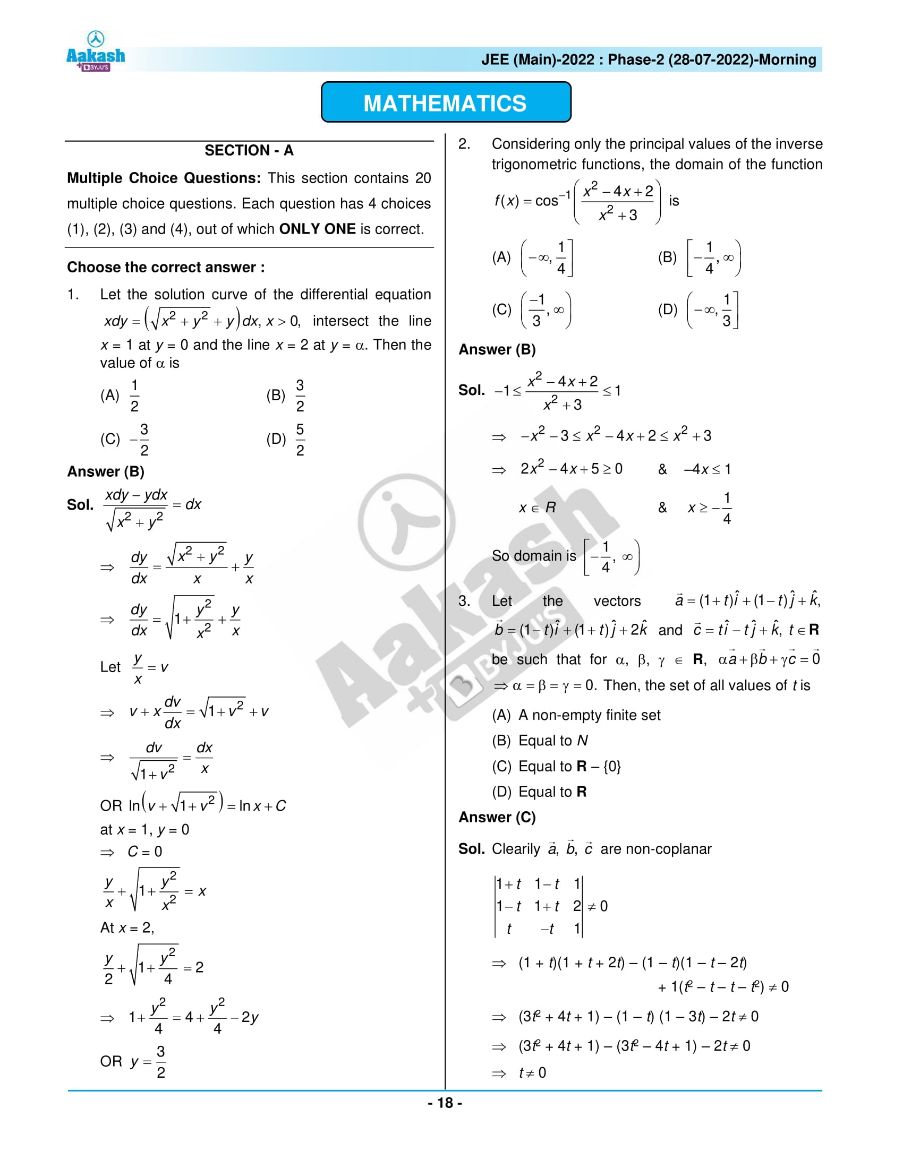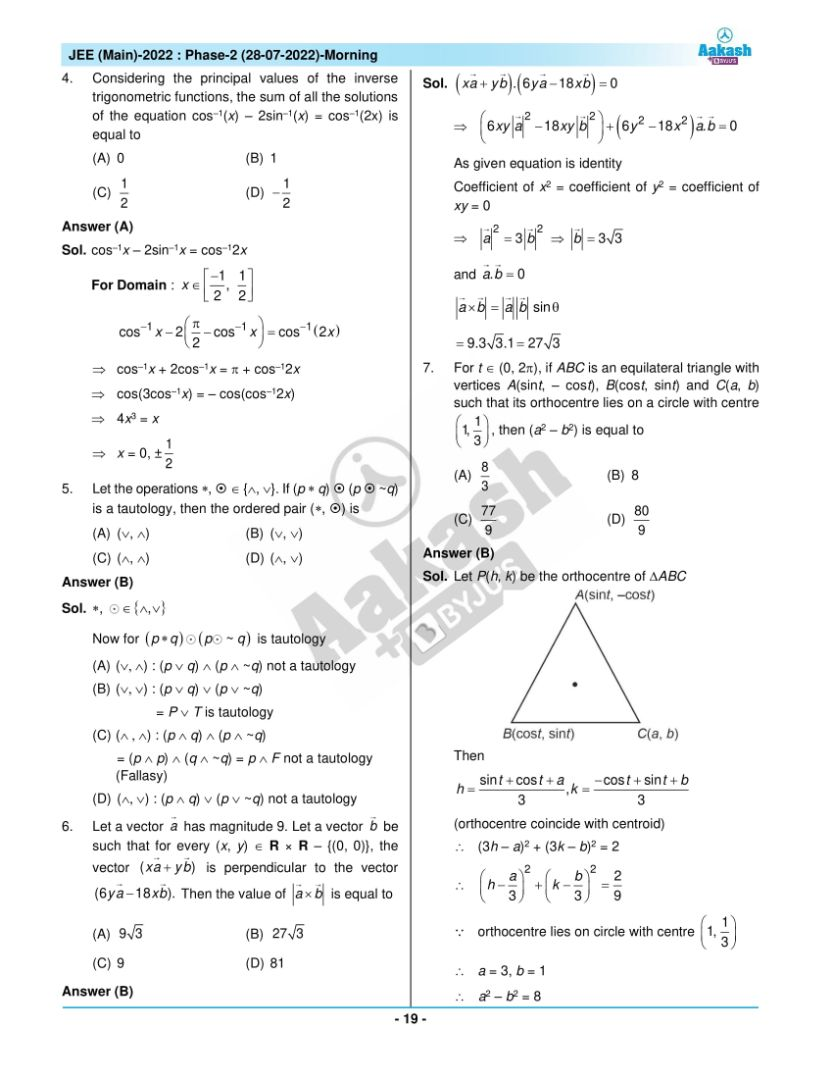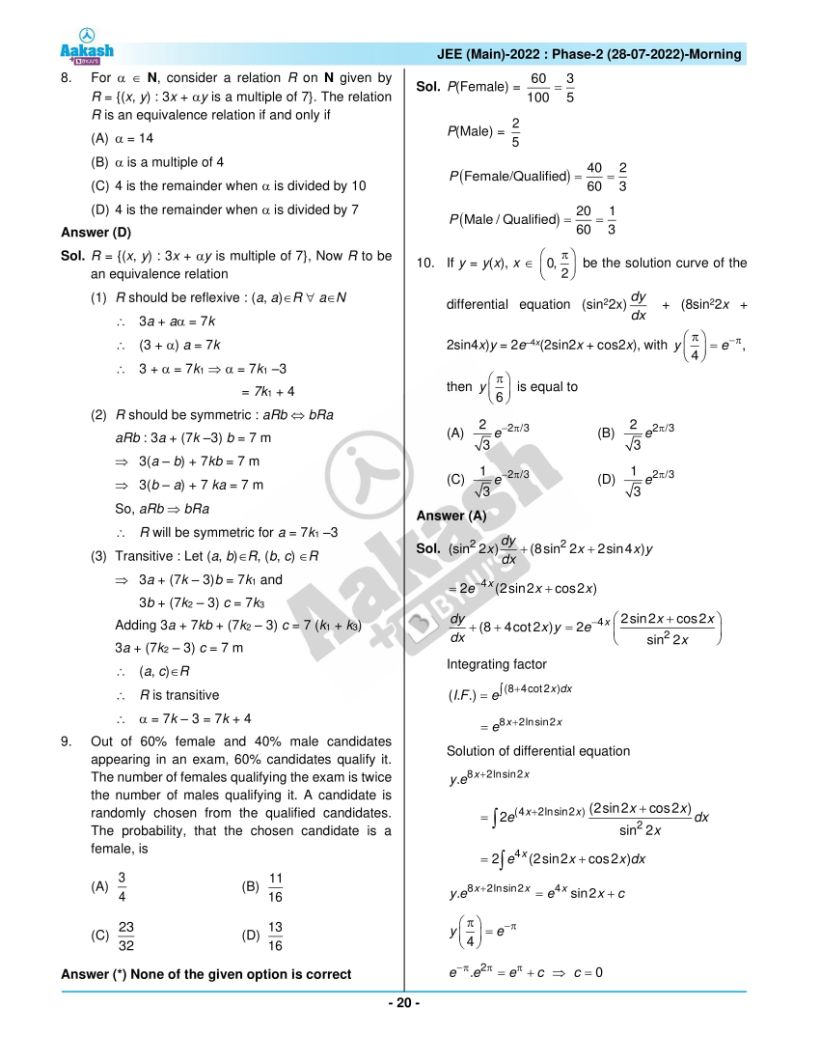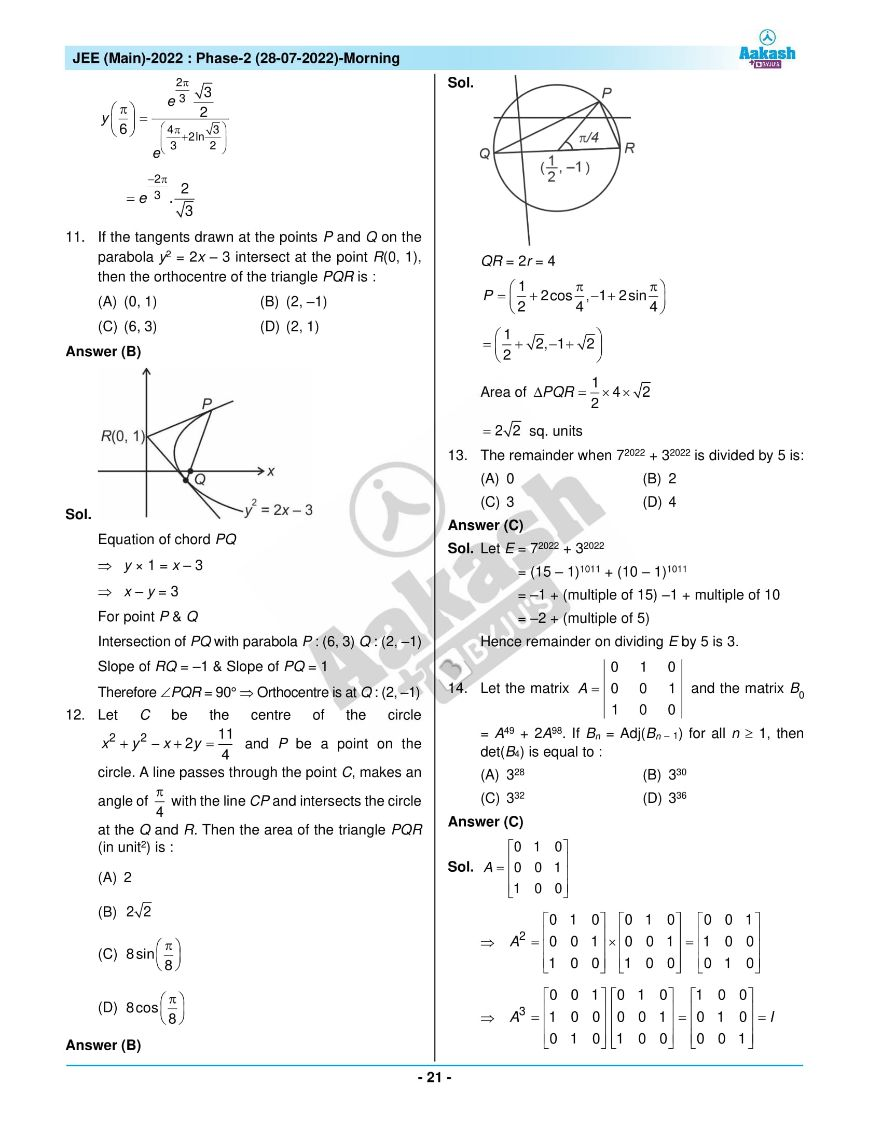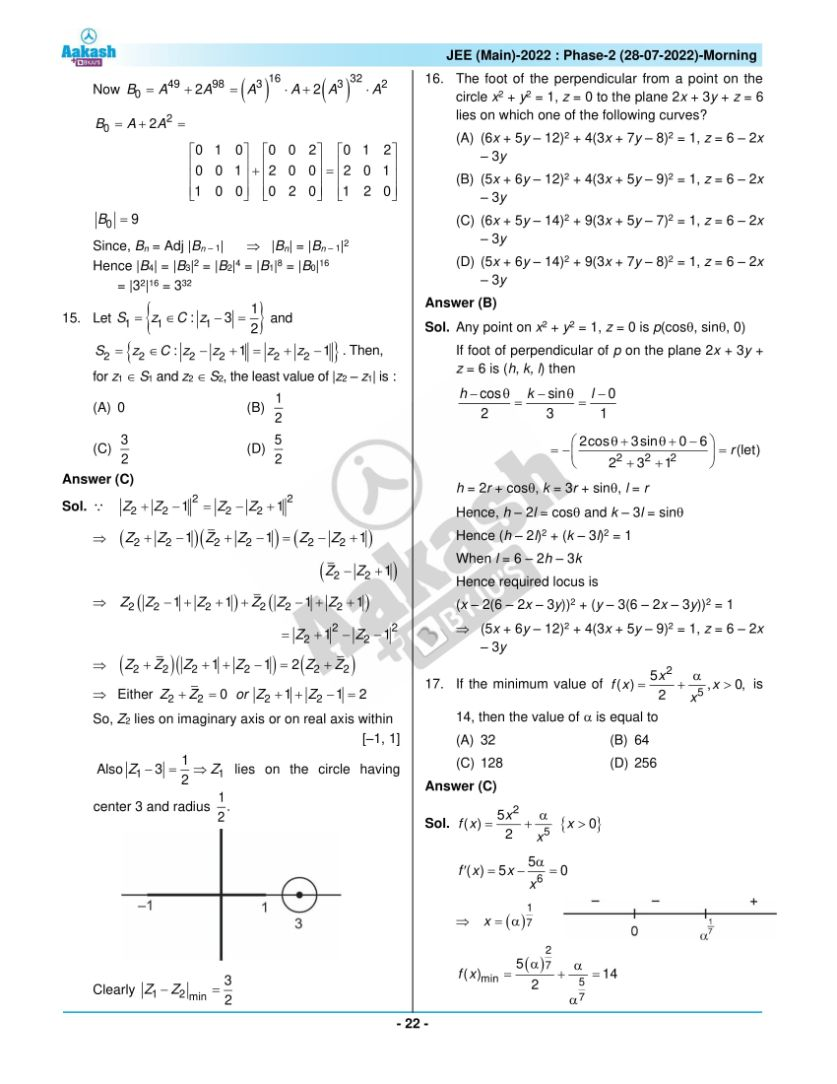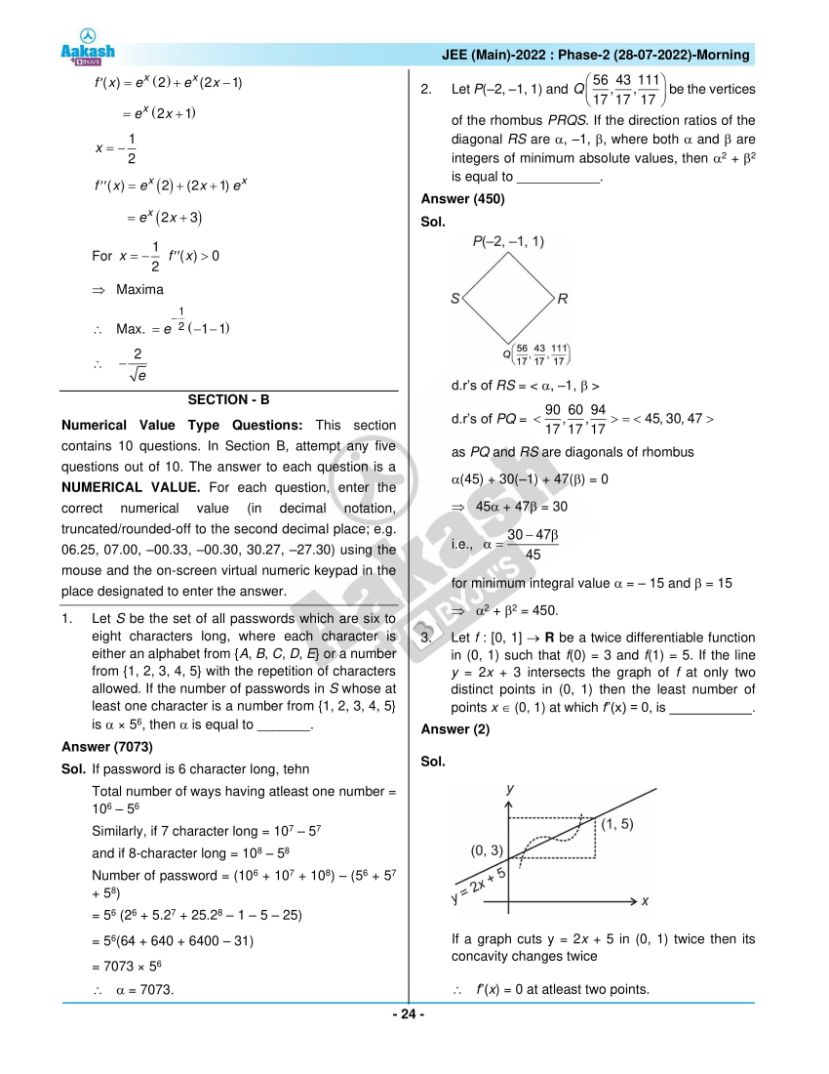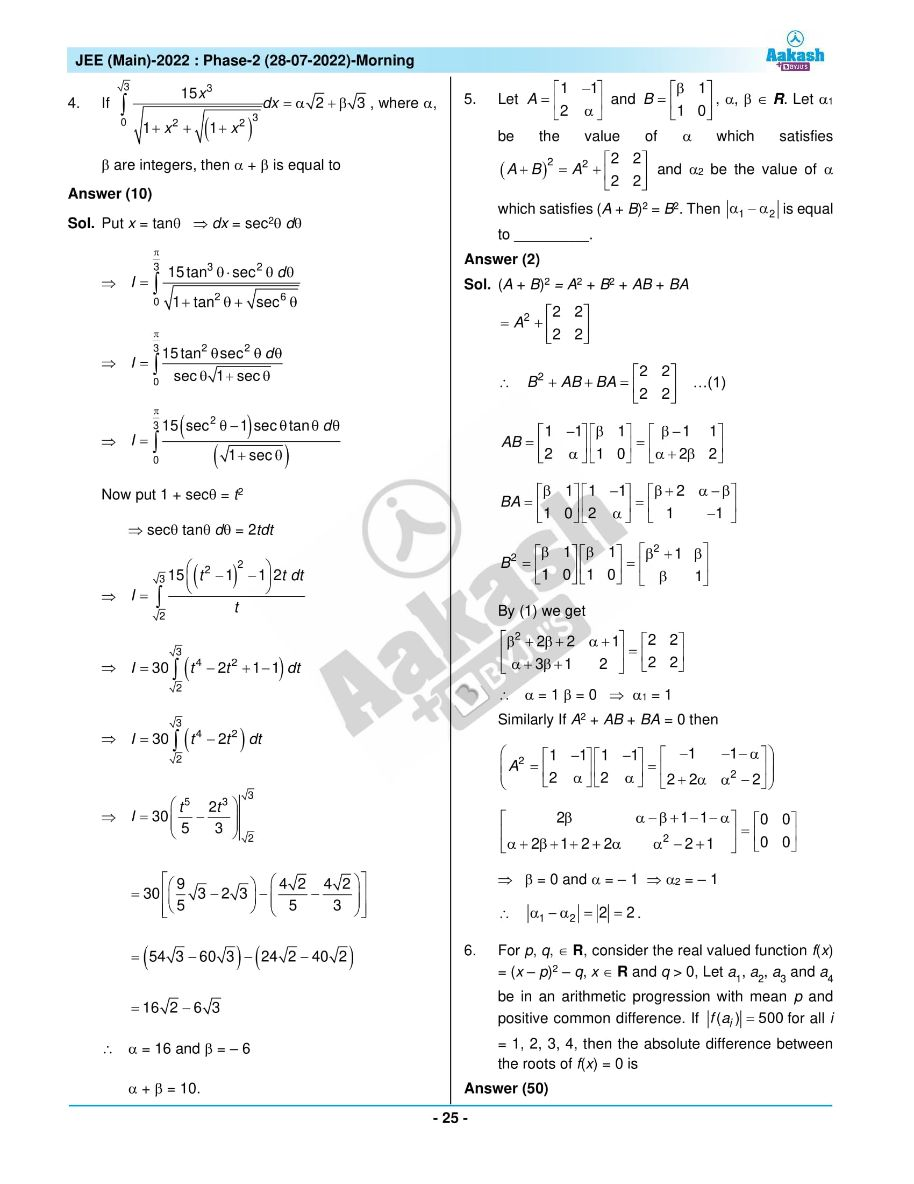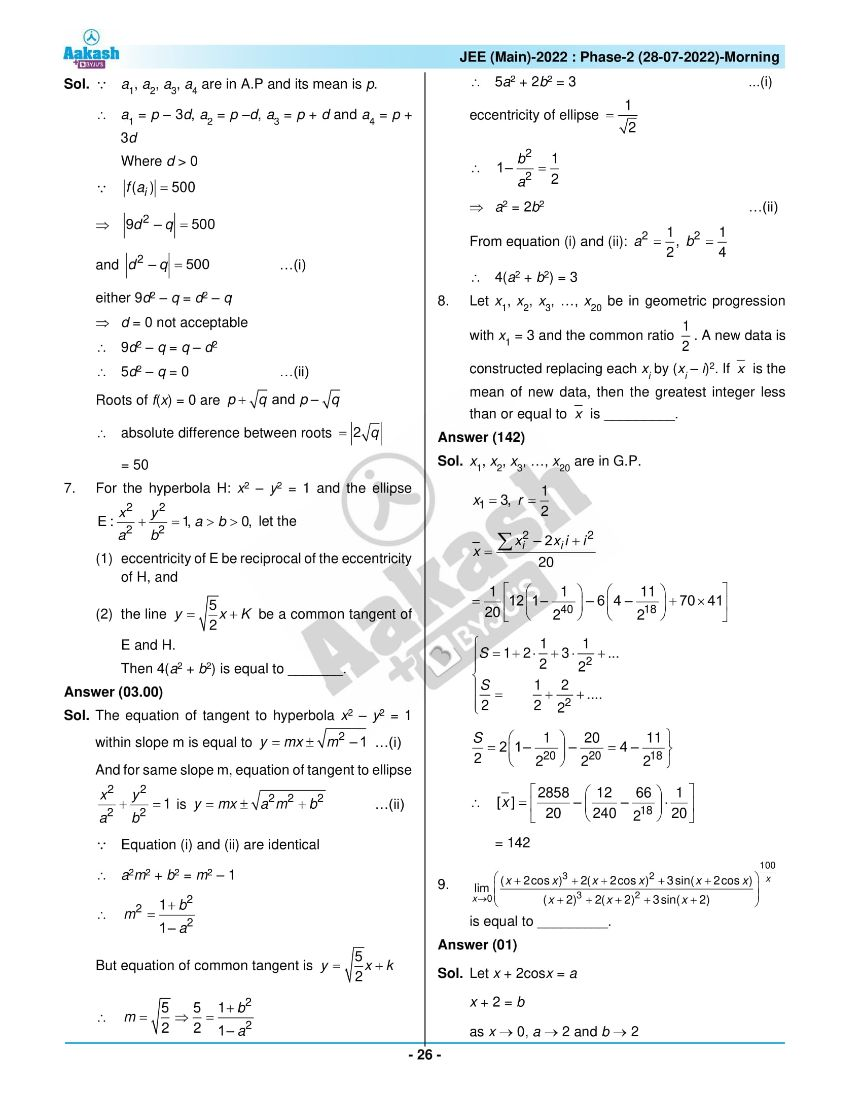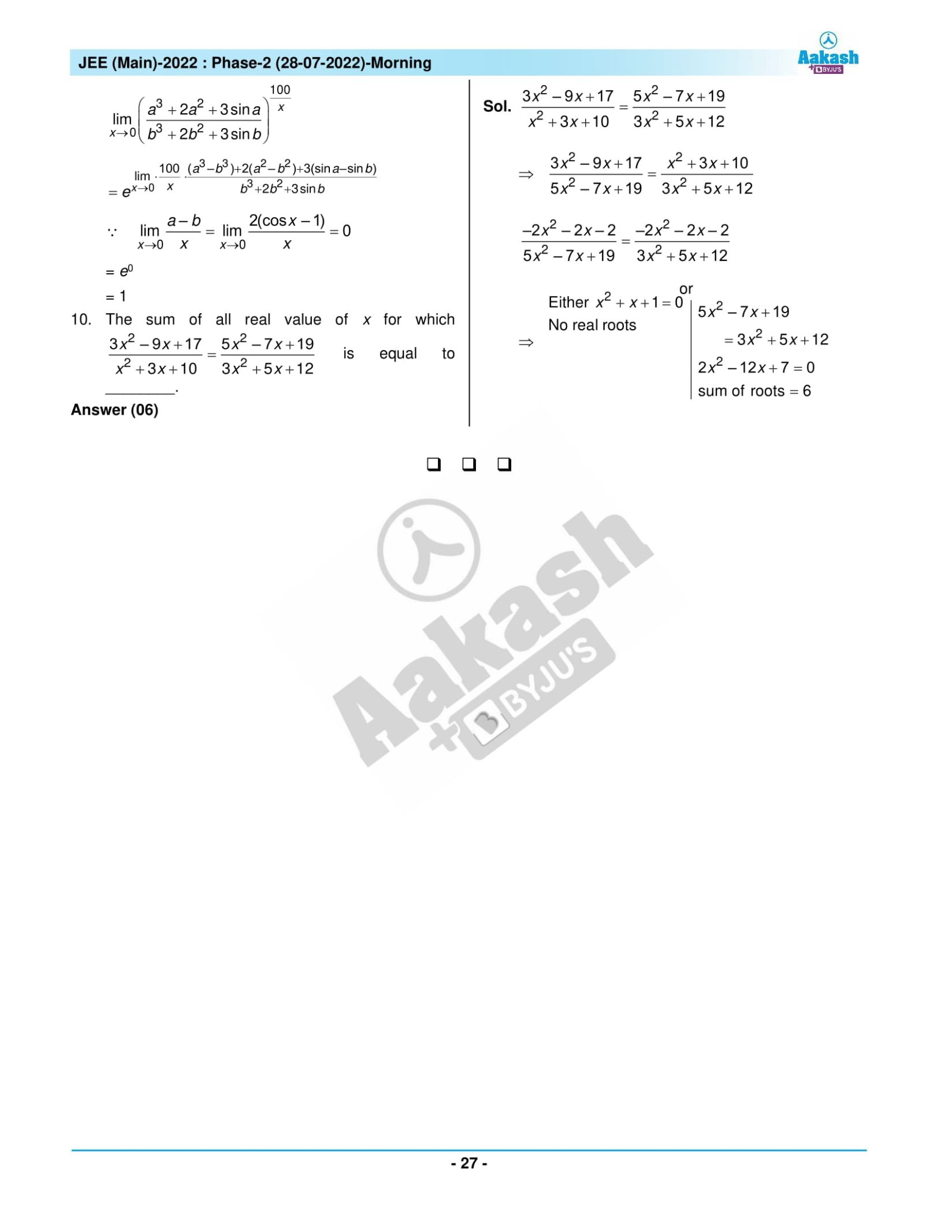## JEE Main 2022 July 28th Shift 1 Paper Analysis